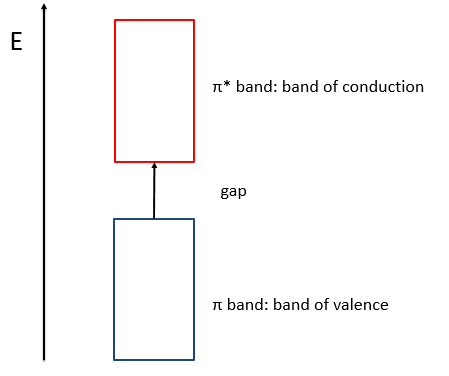# Chapter 14 : MPC – The LCAO theory

This theory says that each molecular orbital Φa is described by a linear combination of atomic orbitals {χ} centred on the M nuclei of the molecule.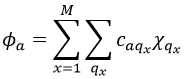The molecular orbitals have the symmetry of one of the irreducible representations of the group G. This symmetry is taken into account in the LCAO coefficients. Some are null (some symmetries are not used) and some are equals in absolute value: only functions of same symmetry interact together to form molecular orbitals.

Given a MO Φa ∈ D(i) of G and the AO {χ}, we can adapt the atomic orbitals to the symmetry of D(i): {χ}à{χ(i)}. Then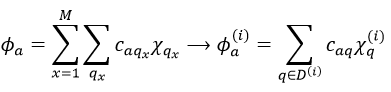The advantage of doing this is that the number n(i) of functions χ(i) in the last expression is smaller than (or equal to) the number n of functions χ in the atomic orbitals. Let’s apply the LCAO theory to an example in which the orthonormal base is composed of two function {Ψ1, Ψ2}, i.e. a case where two states are in interaction with each other.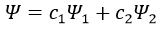The secular determinant is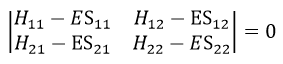As the base is orthonormal, S is a delta of Dirac: S12=S21=0, S11=S22=1 and H12=H21. Posing that the two states H11 and H22 are equidistant from the zero energy, they are separated in energy by 2H: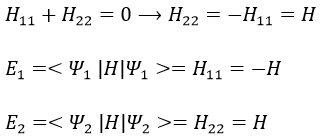We can represent the problem as follow: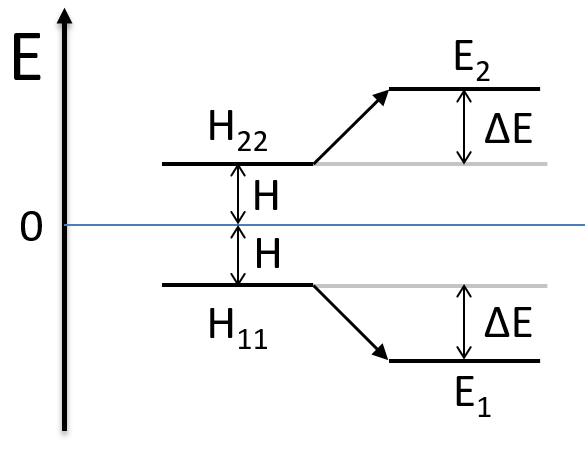The secular determinant is thus reduced to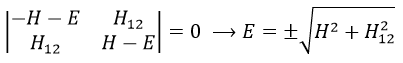and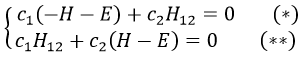If we set c1=1, then c2=(H+E)/H12. The coefficients must still be normed.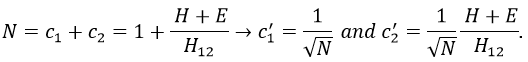Two cases can be considered:

• H=0 with H12<0.

Two degenerated states interact with each other. The result of this interaction is that the states are repelling from each other. One is stabilised and the other one is destabilised by ΔE=H12. We will obtain a bonding state and an antibonding state. H12 is thus a measure of the interaction between the states. The bigger it is, the bigger is the separation between the resulting states. H was the distance between the states that interact together.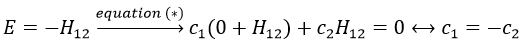Posing that c1=1, then c2=-1. The coefficients must still be normed: c1=1/√2 and c2=-1/√2. As a result, the wave function of the state of energy E=-H12 is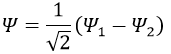We can do the same for the second state (E=H12) and obtain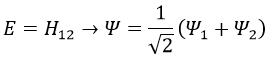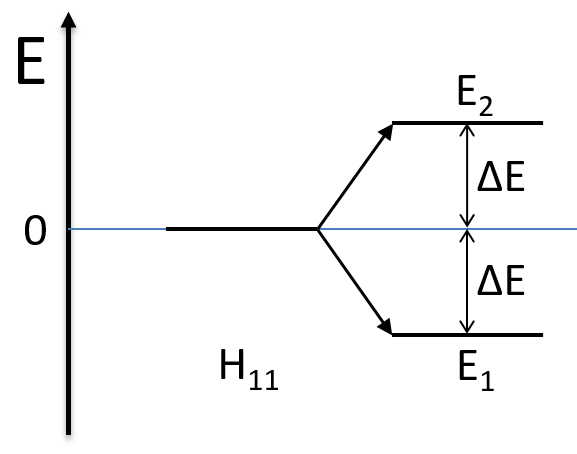• H≠0 and H>>H12

The states that are interacting together have not the same energy. For instance, let’s consider H=2 and H12=-1/2.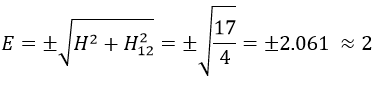The interaction between the two states separated the states but just by a bit. The states did not mix a lot together.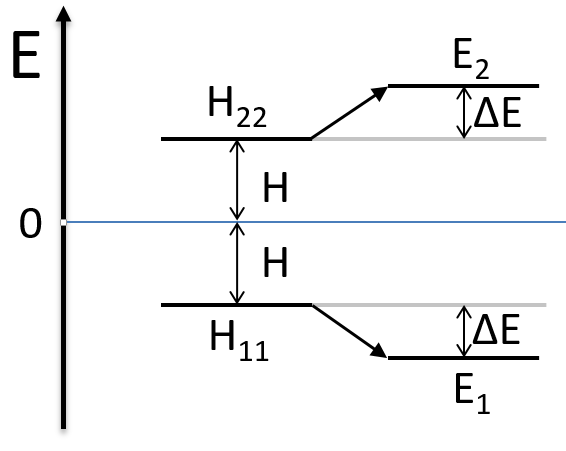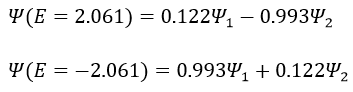The mixing between two states is inversely proportional to the difference of energy between the states.

If H12=0, there is no interaction between the states. It is the case only when the states don’t share any symmetry, i.e. H12 can be different from zero only if Ψ1 and Ψ2 have common proper values with all the operators that commute with Ĥ. As a result, a triplet does not interact with a singlet even if the energies of those states are similar.

Rule of non-cancellation of an integral:

An integral is not equal to zero if the integrand is invariant with regards to all the operation of the group G, i.e. if the reduction of the direct product contains the totally symmetric irreducible representation D(1). In other words, the integral is different from zero if the integrand is totally symmetric.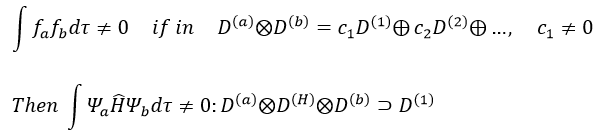Cases of H2+ and H2

The orbitals 1s of the two atoms are interacting together to form molecular orbitals σg and σu. σg is a binding orbital resulting from a constructive interference: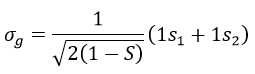Here we considered that the base is not orthonormal. It is why the norm is 1/√(2(1-S)) and not 1/√2. S is the deviation to the orthonormal base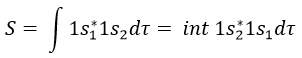In an orthonormal base, S=0. The antibonding state σu is resulting from a destructive interference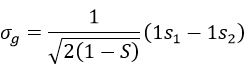The interactions can be seen this way:

When the electron is in a molecular orbital such as the attraction it produces on both nuclei brings the nuclei closer to each other, the effect is binding.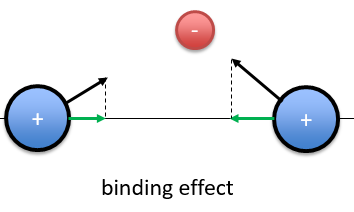In the figure above, the electron is between the two nuclei. The attraction is produces on the nuclei is represented by the black arrows. The green arrows are the projection of the attraction on the axis passing by the two nuclei. The nuclei move thus in the direction of the other nucleus and remain thus together because of the presence of the electron.

If the position of the electron leads to a separation of the nuclei, then the effect is antibonding. The interaction of the electron-nucleus is positive but the intensities and directions are such as the nucleus-nucleus distance increases.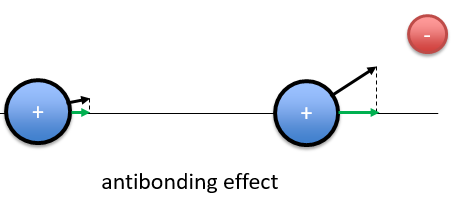In the picture above, both nuclei are attracted by the electron but they move in the same direction with different speeds. The nucleus of the right moves faster than the nucleus of the left and the nuclei move thus away from each other.

The function 1s can be expressed as an exponentially decreasing function centred on the nucleus.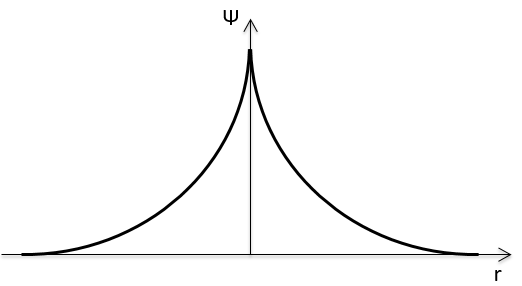We can superimpose the functions 1s of two hydrogen atoms. In the case of σg, the functions add together: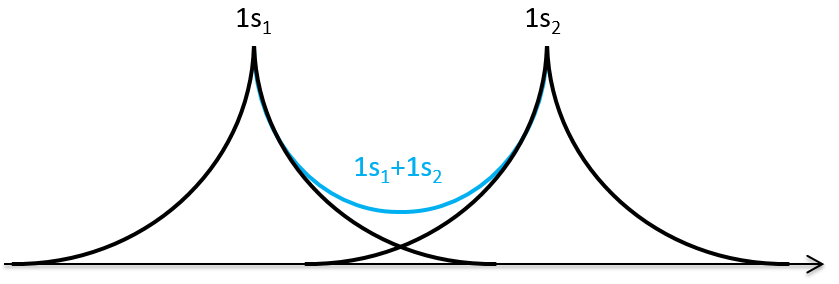We can do the same for σu.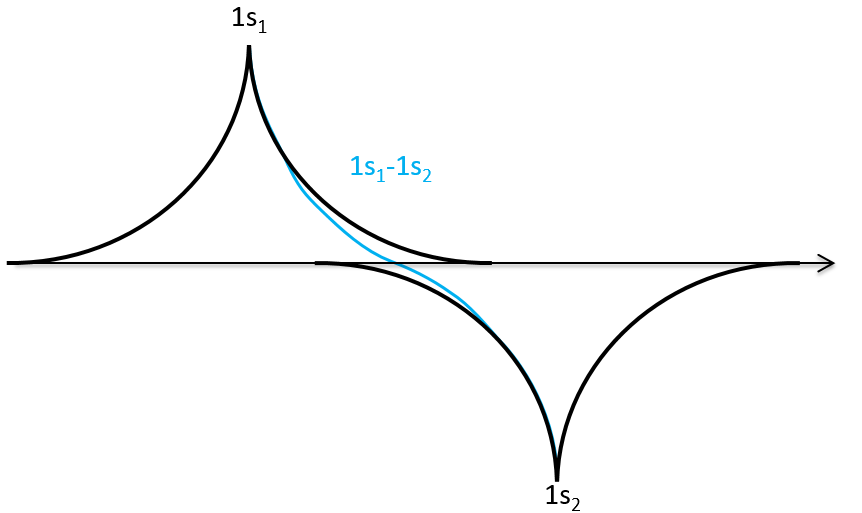If we put those function to the square, we obtain the probability of presence of electrons.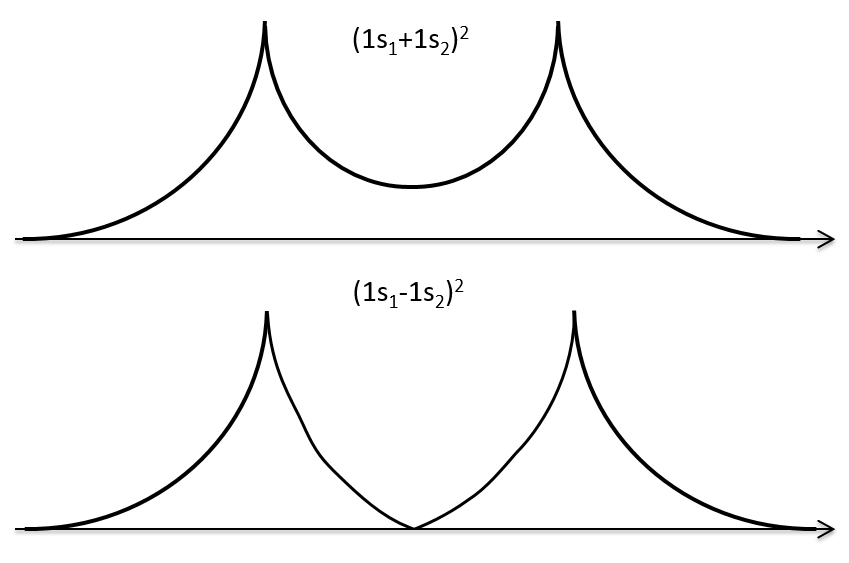In the second case, there is a place between the nuclei where no electron can be found. No liaison can thus be done between the nuclei.

Interaction between 2p orbitals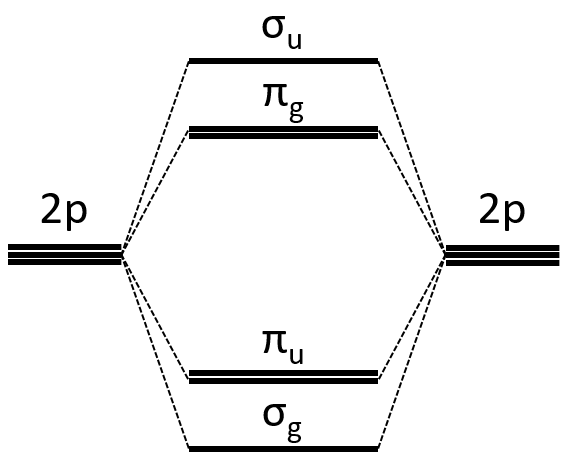All the 2p orbitals don’t interact the same way. The 2pz orbitals interact together to give the σ orbitals. This time, it is not the sum of the atomic orbitals that give the molecular orbital of lower energy and the binding orbital σg.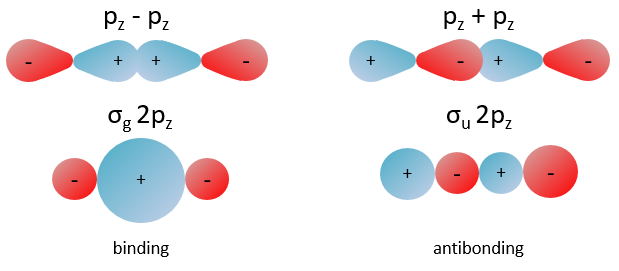The other 2p orbitals (2px, 2py) lead to the π orbitals.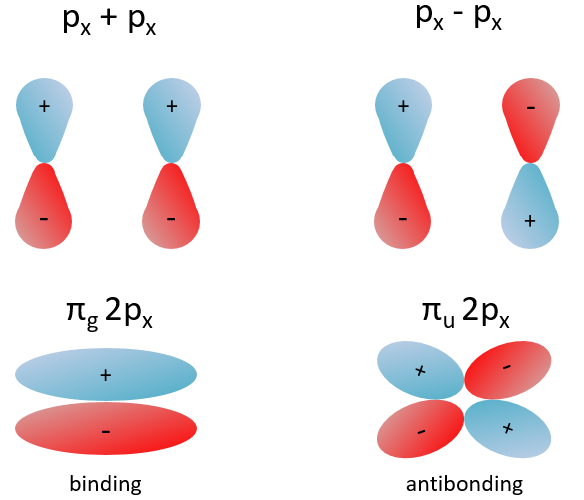Note that the energy of the orbitals depends on the atoms. They all decrease in energy with Z but not with the same speed (see the figure below).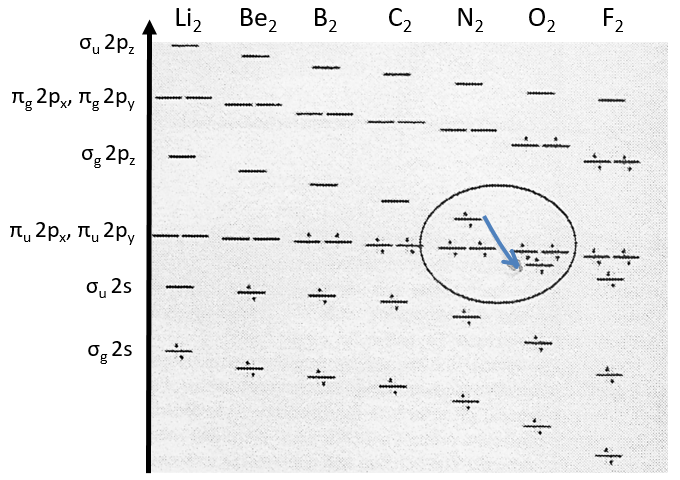The energy of the πu orbitals is almost constant while σg 2px decreases quickly with Z. σg 2px falls under πu at O2.

The energy of the liaison between the two atoms increases up to N2 and decreases after because electrons are placed in antibonding orbitals.

The Walsh diagram

This diagram relates the energies of molecular orbitals of a molecule as a function of the angle that separates the liaisons. It helps to visualise the stability of the liaisons with regards to the symmetry of the molecular orbitals. The following figure shows the Walsh diagram for AH2.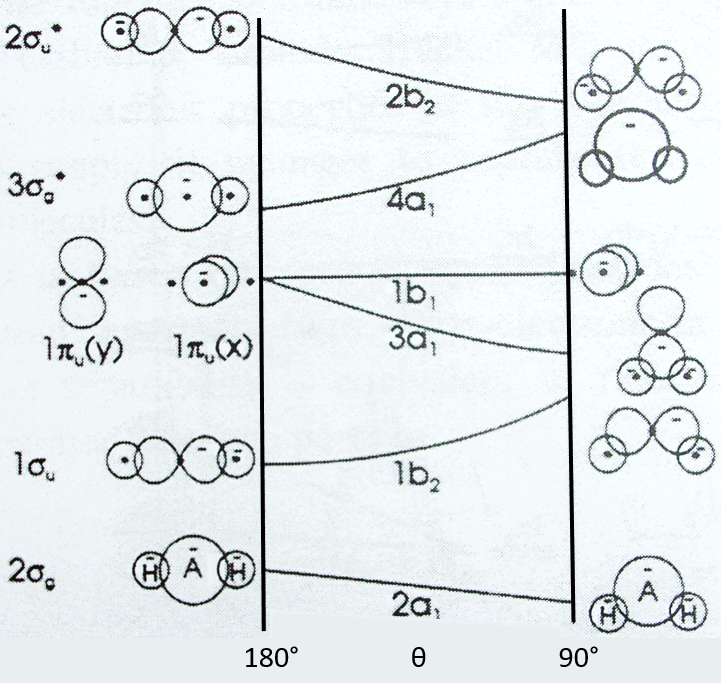On the left one sees the linear molecules. As we go towards the right, the angle between the two liaisons goes towards the right angle, i.e. towards a bent conformation. As the bond angle is distorted, the energy for each of the orbitals can be followed along the lines, allowing a quick approximation of molecular energy as a function of conformation. As we move towards the top, the energy of the liaisons increases. Note that the 1πu orbitals are degenerated for an angle of 180° but separate if we change the conformation of the molecule.

For one molecule, we count the number of electrons of valence. For instance BeH2 has 6 electrons of valence (4 for Be and 1 for each H). We place 2 electrons by line, starting from the bottom (note that the 1a1 line, binding the σg orbitals, is not plotted. The last electrons are on the 1b2 line. One can see that the most stable angle for this molecule is 180°. For BH2 and CH2, the molecules are bent. If one electron is excited, then the conformation of the molecule can change.

Method of Hückel

This method limits the LCAO method to the π electrons. The reason is that a lot of physicochemical properties of the molecules can be explained by the π-π* orbitals. In the method of Hartree-Fock, the secular determinant was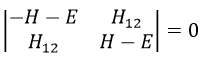We have to solve the determinant for all the orbitals of the molecule, what can quickly become complicated. If we apply the method of Hückel on C2H4 for instance, there is only one π liaison in the molecule and thus only one secular determinant to solve. Considering two degenerated levels of energy α, the secular determinant is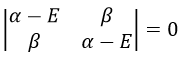α=H11=H22 is the energy of the perpendicular to the plane atomic orbitals of the carbons and beta is the energy of resonance/interaction.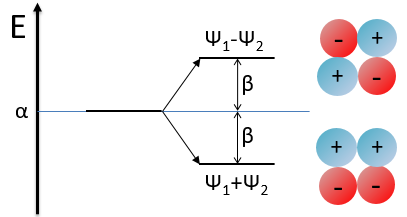The solution found with this method (we won’t do it here) is close to the one obtained with the Hartree-Fock method that consider all of the electrons.

This method can be extended to other systems with π electrons if we pose that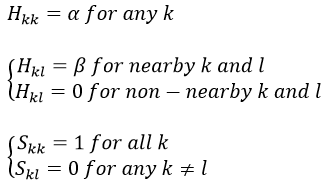If we consider the butadiene, we consider it as the interaction of two π systems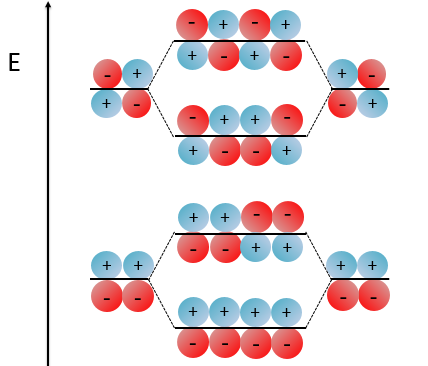With the increase of π electrons, there are more binding states and one can see that, looking from the bottom to the top, the organisation of the orbitals follows a simple rule: the number of times that the signs are reversed increases by one at each orbital. One talk about the “wavenumber” of the orbitals. Indeed, on the lowest energy state, all the orbitals are aligned. There is no change of orientation of the orbital. On the second lowest state, the two orientations of the orbitals are present but they are grouped. There is only one change of orientation. The third level has 2 changes of orientations, there are 3 changes on the fourth lowest state, 4 on the fifth, etc… If we look at the orbitals as a wave, the wavenumber is indeed increasing with the energy of the state.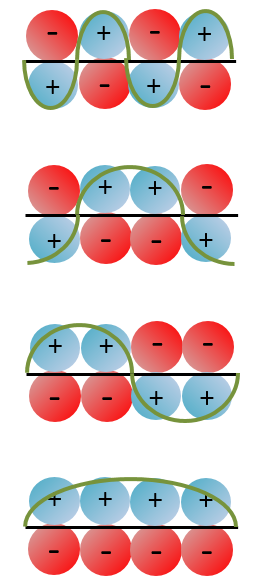When the amount of π liaisons increases,

• the separation in energy between the states of same type (binding or antibonding) decreases and for a large amount of liaisons (in polymer for instance), we talk about a band of valence for the block of binding states and about a band of conduction for the block of antibonding states, separated by a gap.
• the amount of states increases in both bands,
• the separation in energy between the band of valence and of conduction decreases.